# How to Implement PIVOT and UNPIVOT in SQL Server

## How to Implement PIVOT and UNPIVOT in SQL Server

In this article, I am going to discuss How to Implement PIVOT and UNPIVOT in SQL Server with examples. Please read our previous article where we discussed Change Data Capture in detail. The PIVOT and UNPIVOT are two operators in SQL Server that are basically used to generate multi-dimensional reports. The PIVOT operator is used when you want to transfer the row-wise data into column-wise and the UNPIVOT operator is used when you want to convert the column-wise data into row-wise. Before implementing pivot and unpivot, let us first understand what exactly pivot means.

##### What is the Pivot Operator in SQL Server?

In order to understand this, we are going to use the following Customers table. As you can see, the following Customers table having three columns (CustomerName, ProductName, and Amount). The following table tells that which customer brought which products. Some customers bought both laptops and Desktop while some customers bought either laptop or desktop. Again a few customers are there, they bought a product multiple times.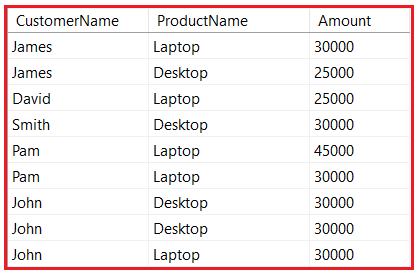Please execute the below SQL Script to create and populate the Customers table with the required data.

```CREATE TABLE Customers
(
CustomerName VARCHAR(50),
ProductName VARCHAR(50),
Amount INT
)
GO

INSERT INTO Customers VALUES('James', 'Laptop', 30000)
INSERT INTO Customers VALUES('James', 'Desktop', 25000)
INSERT INTO Customers VALUES('David', 'Laptop', 25000)
INSERT INTO Customers VALUES('Smith', 'Desktop', 30000)
INSERT INTO Customers VALUES('Pam', 'Laptop', 45000)
INSERT INTO Customers VALUES('Pam', 'Laptop', 30000)
INSERT INTO Customers VALUES('John', 'Desktop', 30000)
INSERT INTO Customers VALUES('John', 'Desktop', 30000)
INSERT INTO Customers VALUES('John', 'Laptop', 30000)
```

Let us visualize the above customer’s data from a different angle. For example, we want to tell how many customers bought laptops and how many customers bought Desktop as shown in the below image. Here, actually, we have to change the perspective of row-wise data into column-wise.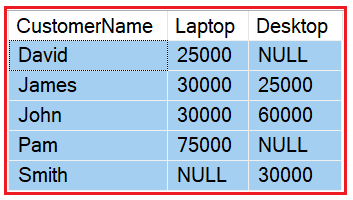So, basically, we want to convert the row-wise data into column-wise. Now, the question is how we can implement this in SQL Server? The SQL Server provides one built-in function called Pivot which we can use to change the row-wise data into column-wise.

##### How to implement PIVOT in SQL Server?

Let us understand how to implement the PIVOT operator in SQL Server. In order to understand Pivot, please have a look at the following image. As you can see in the below image, we have divided the Pivot code into three sections.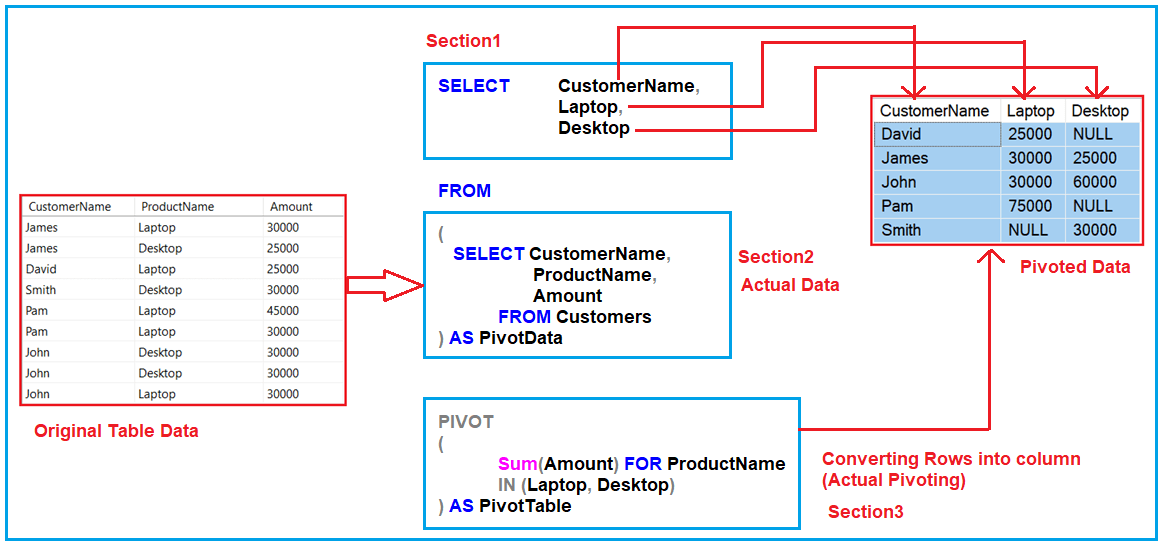###### Section1:

Section 1 contains a select statement and this select statement has the column name that we want to display. In the output, we want CustomerName, Laptop, and Desktop and hence the select statement contains these three columns. The first column (Customername) is the non-pivot column and the rest are pivot columns (Laptop and Desktop).

###### Section2:

This section actually gets the actual data that is needed for the pivot report. In our example, the actual data is nothing but the Customers table data. Hence, you can see, here we use a select statement to get the data from the Customers table.

###### Section3:

This is the section where your Pivot function lies. Within the function first, we need to use an aggregate method like SUM, Count, etc. Here, we are using the SUM aggregate method and to this method, we pass the Amount filed as we are performing sum on the Amount column. In the For clause, we need to pass the column name which contains values that are going to be column header. In our case, it is the ProductName column. In the IN clause, we need to specify the Pivoted column names. In our example, it is a Laptop and Desktop.

###### Example: PIVOT Operator

Let us execute the following code and see the output as expected.

```-- Section1: Define the column names
SELECT	CustomerName,
Laptop,
Desktop
FROM

-- Section2: Get the Actual Data
(
SELECT  CustomerName,
ProductName,
Amount
FROM Customers
) AS PivotData

-- Section3: Pivot function
PIVOT
(
Sum(Amount) FOR ProductName
IN (Laptop, Desktop)
) AS PivotTable
```

The following is the syntax of the Pivot Operator.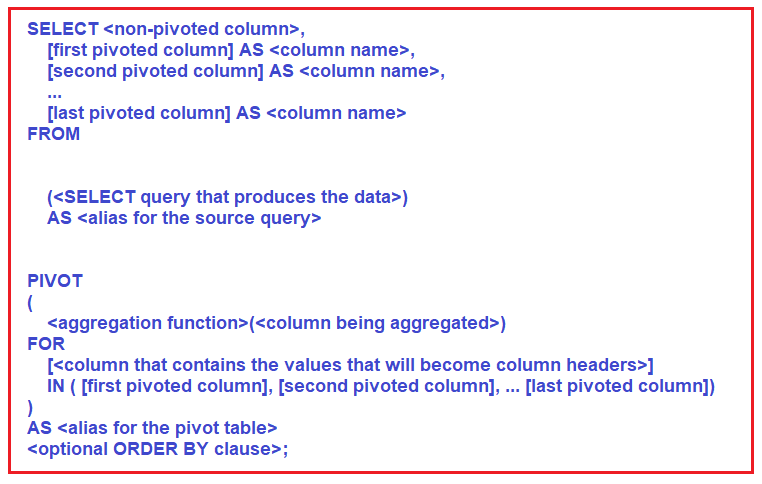##### UNPIVOT in SQL Server:

The UNPIVOT operator performs exactly the opposite operation to PIVOT. That is, the UNPIVOT operator turns COLUMNS into ROWS. Let us understand this with an example. We are going to use the following ProductSales table to understand this concept.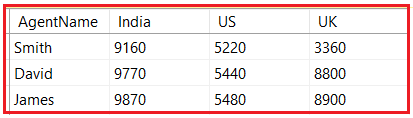Please use the below SQL Script to create and populate the ProductSales table with the required data.

```Create Table ProductSales
(
AgentName VARCHAR(50),
India int,
US int,
UK int
)
Go

INSERT INTO ProductSales VALUES ('Smith', 9160, 5220, 3360)
INSERT INTO ProductSales VALUES ('David', 9770, 5440, 8800)
INSERT INTO ProductSales VALUES ('James', 9870, 5480, 8900)
Go
```

Let us visualize the above Product Sales data from a different perspective. For example, we want to tell the sales amount, per count, per agent as shown below. Here, actually, we have to change the perspective of column-wise data into row-wise.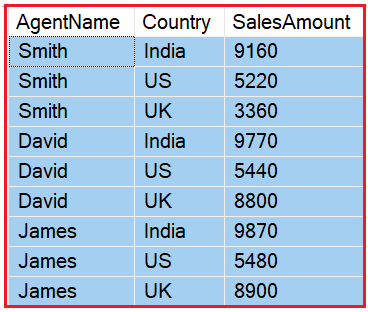Here, we need to convert the column-wise data into row-wise. Now, the question is how we can do this in SQL Server? The SQL Server provides another built-in function called UNPIVOT which we can use to change the column-wise data into row-wise.

##### How to implement UNPIVOT in SQL Server?

Let us understand how to use UNPIVOT operator in SQL Server. In order to understand how to use UNPIVOT; please have a look at the following diagram. As you can see in the below image, like PIVOT, here we also have divided the UNPIVOT code into three sections.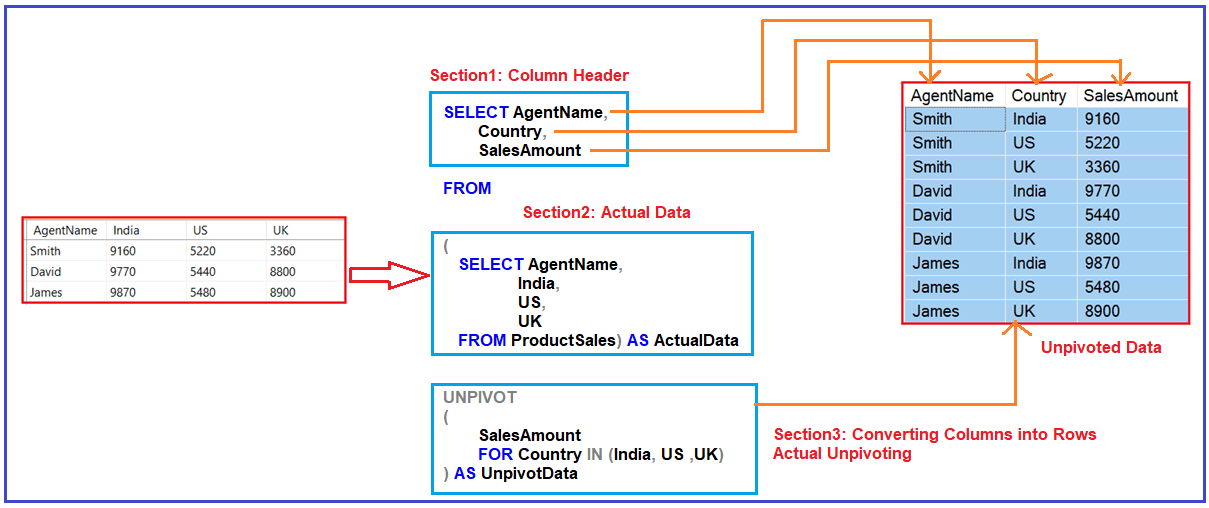###### Section1:

Section 1 contains a select statement and this select statement has the column name that we want to display in the pivot report. In the output, we want AgentName, Country, and SalesAmount and hence the select statement contains these three columns. The first column (AgentName) is the normal column and the rest are unpivot columns (Country and SalesAmount).

###### Section2:

This section gets the actual data from the actual table which is needed for the unpivot report. In our example, the actual data is nothing but the ProductSales table data. Hence, you can see, here we use a select statement to get the data from the ProductSales table.

###### Section3:

This is the section where our UNPIVOT function works. As we want sales amount by country, so we use SalesAmount For Country option here. As we want the column values India, US, UK as the column header, so here we pass these column values to the IN clause.

##### Example: UNPIVOT Operator

Please execute the following SQL Script and see the output.

```SELECT AgentName, Country, SalesAmount

FROM

(
SELECT AgentName,
India,
US,
UK
FROM ProductSales) AS ActualData

UNPIVOT
(
SalesAmount
FOR Country IN (India, US, UK)
) AS UnpivotData
```

That’s it for today. In the next article, I am going to discuss How to Reverse the PIVOT table in SQL Server with examples. Here, in this article, I try to explain How to Implement PIVOT and UNPIVOT in SQL Server. I hope you enjoy this How to Implement PIVOT and UNPIVOT in SQL Server article and understand the need and use of PIVOT and UNPIVOT in SQL Server.